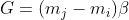# Balancing data sets with Crucio ADASYN

Nowadays the majority of data sets in the industry are unbalanced. Meaning that a class has a higher frequency than others. Very often classifiers in such cases due to the unbalance of the data predict all samples as the most frequent class. To solve this problem we decided at Sigmoid to create a package that will have implemented all oversampling methods. We named it Crucio, and in this article, I will tell you about ADASYN(Adaptive Synthetic).

## How does the ADASYN work?

Firstly we must calculate the number of samples to generate.mj — # of majority samples, mi — # of minority samples.

Beta is a hyperparameter of the ADASYN between 0 and 1. So if it is set up to 1 then the classes will be perfectly balanced.

Now for each minority sample, we should calculate the r-value. For this, we should find the K-nearest neighbors of every minority sample. Then r-value for each minority sample is calculated as the ratio of the number of the majority samples in the neighborhood. After this r-values should be normalized.

Now, this vector of r-values should be multiplied by G. We get an updated vector G, which we will use to find out how many samples we should generate for each minority sample. That’s why we normalized the r vector. By multiplying it to G, G became a vector with the sum equal to the number of samples that should be generated. Also, every element of this vector, as I said previously, represent how many samples should we generate for these certain samples. The bigger the number of majority samples, in the neighborhood, the more samples we will generate there.

In such a way we will not generate samples in the middle of the minority sample vector space, but only of its border. That's where the name “Adaptive” comes from.

The last steps include the generation of the new samples. By iterating the G vector, for every sample, we will use its Gi value to decide how many values we will generate. For the generation of the new samples, ADASYN chooses from every neighborhood 2 minority samples. The new samples is generated using the formula below.

Lambda here is a random number between 0 and 1.

In case you didn’t install crucio yet, then type in the terminal the following:

``````pip install crucio
``````

Now we can import the algorithm and create the ADASYN balancer.

``````from crucio import ADASYN

``````

ADASYN constructor has the following parameters:

• binary_columns (list, default=None) : if set to then it will check if the listed columns are in the passed data frame then they will be transformed to binary ones after generating new points.
• beta (float, default = 1.0) : used when the number of minority samples to generate is calculated.
• k (int, default = 5) : the number of neighbors that ADASYN should find for every minority class.
• seed (int, default = 42) : seed for random number generation.

The balance function takes only 2 parameters:

• df (pd.DataFrame) : the pandas data frame that should be balanced.
• target (str) : the name of the column from the data frame that should be balanced.

Now let’s apply it to a data set. We will use it on the Pokemon dataset. The target column (Legendary) is unbalanced, only 8% is not legendary.
We recommend you, before applying any module from Crucio, to first split your data into a train and test data sets and balance only the train set. In such a way you will test the performance of the model only on natural data.
The results before balancing and after.

As we can see the samples generated by ADASYN are more conservative than the ones generated by the ICOTE for example. Also, it slightly helps out the model by making the accuracy higher.